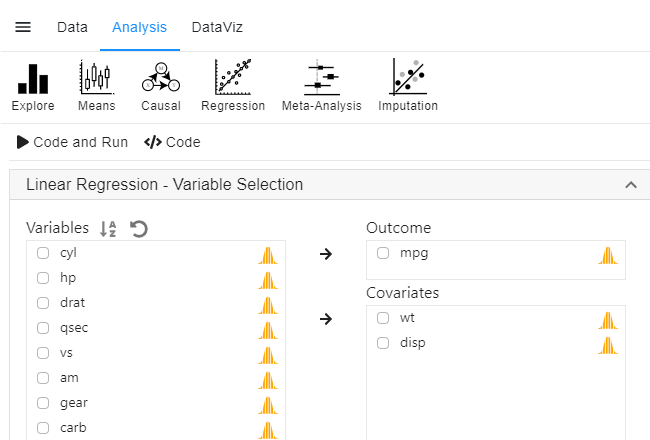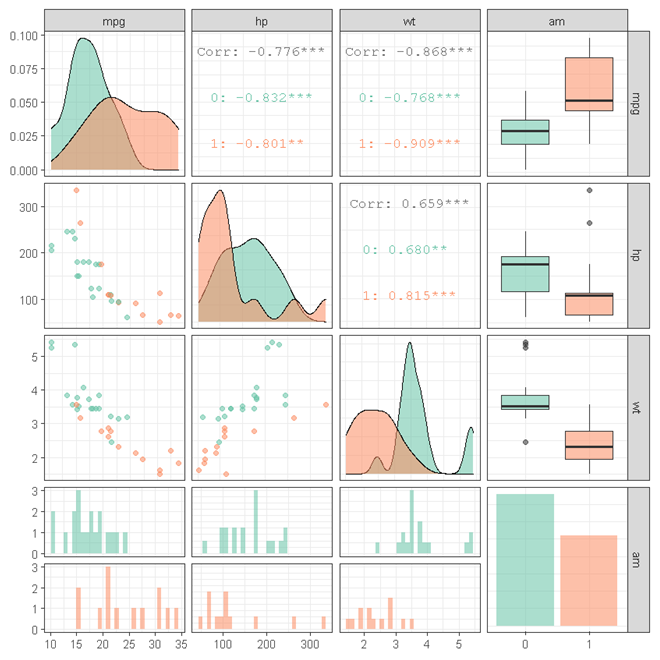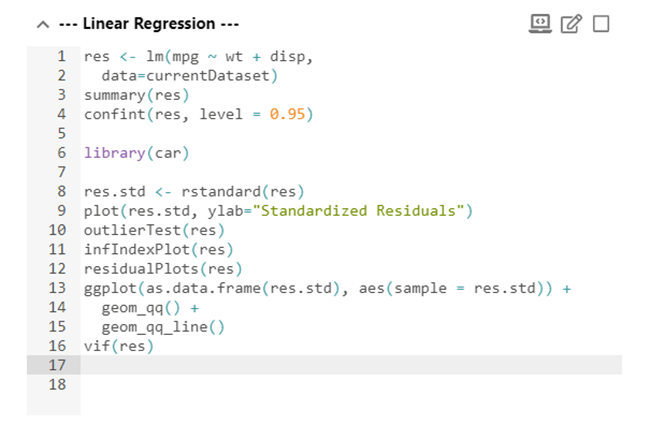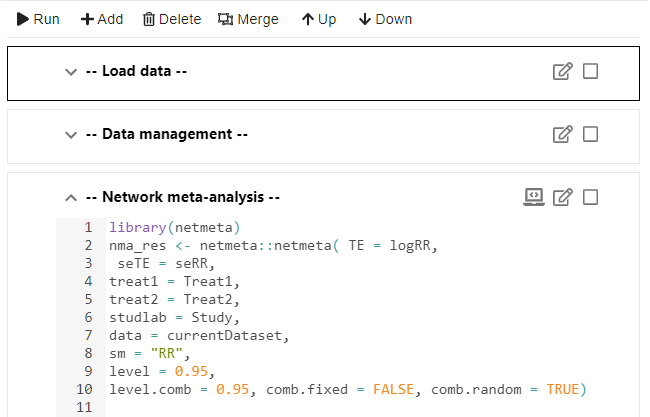StatsNotebook is a full featured open source statistical package. Its design focuses on Simplicity, Functionality and Reproducibility.StatsNotebook provides simple interface for a large range of analyses and data visualisation. Most of analyses can be completed with just a few clicks.

The following analyses are currently supported in StatsNotebook, and Rexi will ensure that the list will keep growing…

• Independent/ Dependent-sample T-test
• ANOVA/ ANCOVA
• Linear regression
• General/ Generalised linear model
• Logistic regression
• Poisson regression
• Negative binomial regression
• Multinomial logistic regression
• Generalized linear mixed model/ Multilevel model
• Mixed-effect regression (multilevel regression)
• Mixed-effect logistic regression (multilevel logistic regression)
• Mixed-effect Poisson regression (multilevel Poisson regression)
• Robust regression/ Mixed-effect robust regression
• Causal mediation analysis
• Meta-analysis/ Meta-regression/ Network Meta-analysis
• Multiple imputationThe following plots can be easily made in StatsNotebook with ggplot2.

• Density plot
• Histogram
• Boxplot/ Violin plot
• Bar graph
• Scatterplot with line of best fit
• Correlogram
• Line graphStatsNotebook is fully integrated with R, the most powerful statistical language with a vibrant community of statistics gurus. The Notebook interface is a R console in disguise! You can interact directly with R and run any R codes in the Notebook interface.StatsNotebook is designed for reproducible research. R codes are arranged in blocks in the notebook interface. Analyses can be re-run and results regenerated any time.

Dr. Gary Chan, Co-founder
Dr. Janni Leung, Co-founder
–UNIDENTIFIED–, Co-founder
Ms. Carmen Lim
Ms. Tianze Sun
Dr. Daniel Stjepanović